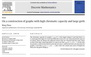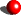Bing Zhou

Professor of Mathematics
Department of Mathematics
Trent University

 A picture My academic ancestors How to contact me

 Research My main areas of research are in graph theory and combinatorics. I am particularly interested in graph coloring, extremal problems involving chromatic numbers and planar graphs. I have also done some work in hypergraphs and partially ordered sets.Some of my recent papers: 1. On a construction of graphs with high chromatic capacity and large girth, in Discrete Mathemaitcs. 2. A lower bound for the chromatic capacity in terms of the chromatic number of a graph, in Discrete Mathematics. 3. On graph orientation and the number of vertices in a longest directed path, submitted. 4. On color critical graphs with large adaptable chromatic numbers, submitted.

 Teaching 2015 FallMATH 1110H   Calculus IMATH 4620H   Graph Theory 2016 WinterMATH 1120H   Calculus IIMATH 3610H   Discrete optimization